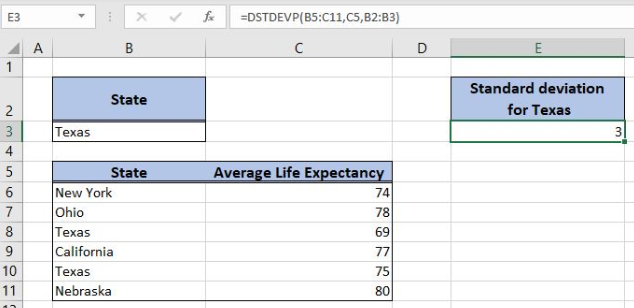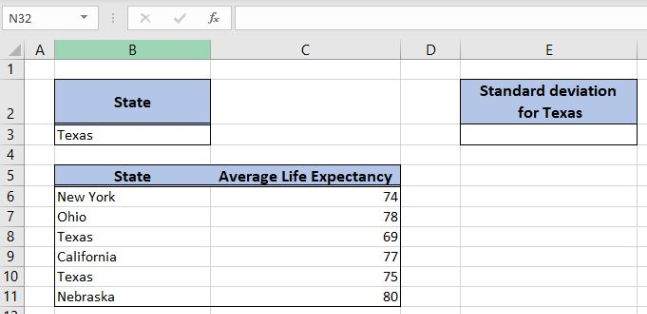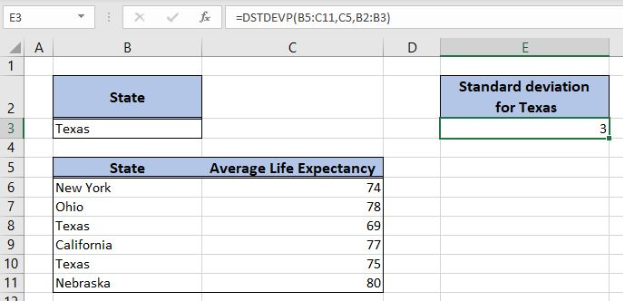Get instant live expert help with Excel or Google Sheets“My Excelchat expert helped me in less than 20 minutes, saving me what would have been 5 hours of work!”

#### Post your problem and you'll get expert help in seconds

Your message must be at least 40 characters
Our professional experts are available now. Your privacy is guaranteed.

# How to Use the Excel DSTDEVP Function

Excel allows a user to calculate the standard derivation using the DSTDEVP functions. This step by step tutorial will assist all levels of Excel users in calculating the standard deviation for the population.Figure 1. The result of the DSTDEVP function

## Syntax of the DSTDEVP Formula

The generic formula for the DSTDEVP function is:

`=DSTDEVP(database, field, criteria)`

The parameters of the DSTDEVP function are:

• database – a database with values for standard deviation
• field – an index of column or field name in a database for the value field
• criteria – a table containing conditions. This table must have the same field names as the database.

## Setting up Our Data for the Formula

Let’s look at the structure of the data we will use. In the range B5:C11, we have the database with columns “State” and “Average Life Expectancy”. In the range B2:B3, we have the criteria. In the cell E3, we want to get the standard deviation for the criteria.Figure 2. Data that we will use in the example

## Calculating the Standard Deviation Using the DSTDEVP Formula

In our example, we want to calculate the standard deviation of the average life expectancy for Texas. The result will be in the cell E3.

The formula looks like:

`=DSTDEVP(B5:C11, C5, B2:B3)`

The parameter database is the range B5:C11. The field parameter is the cell C5. We could also put the name (“Average Life Expectancy”) or 2, as the index of the column in the database. The criteria parameter is B2:B3.

To apply the DSTDEVP formula, we need to follow these steps:

• Select cell E3 and click on it
• Insert the formula: `=DSTDEVP(B5:C11, C5, B2:B3)`
• Press enter.Figure 3. Using the formula to calculate the standard deviation

As we can see in Figure 3, the standard deviation for Texas, based on two values in the database is 3. Therefore, the result of the DSTDEVP function in the cell E3 is 3.

Most of the time, the problem you will need to solve will be more complex than a simple application of a formula or function. If you want to save hours of research and frustration, try our live Excelchat service! Our Excel Experts are available 24/7 to answer any Excel question you may have. We guarantee a connection within 30 seconds and a customized solution within 20 minutes.

### Did this post not answer your question? Get a solution from connecting with the expert.Another blog reader asked this question today on Excelchat:## Subscribe to Excelchat.coAnother blog reader asked this question today on Excelchat: